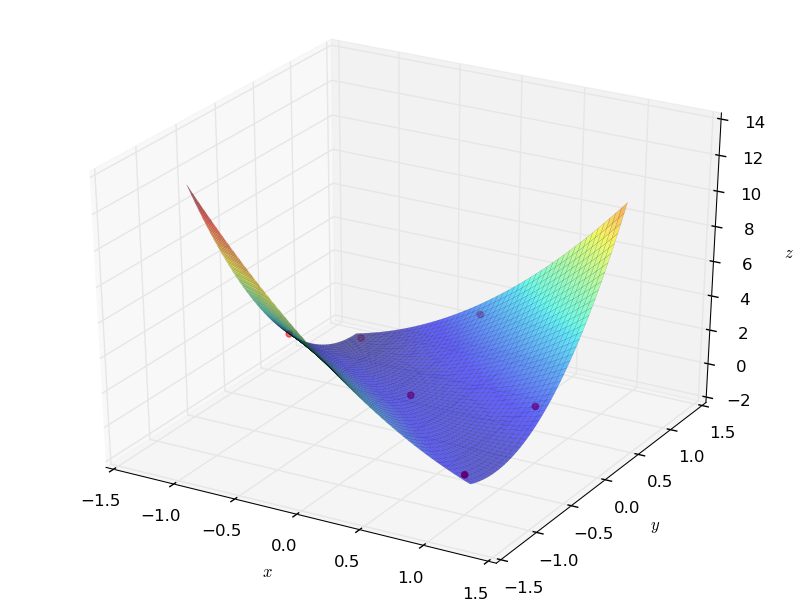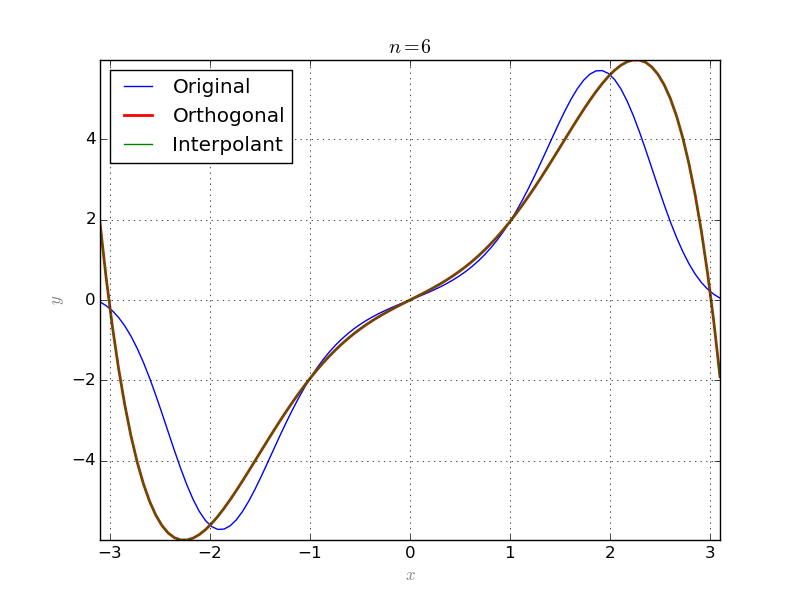# Interpolation¶

## Lagrange interpolation¶

Suppose that a list of $$m+1$$ points $$\{(x_0, y_0),\dots,(x_{m}, y_{m})\}$$ in $$\mathbb{R}^2$$ is given, such that $$x_i\neq x_j$$, if $$i\neq j$$. Then

$p({\bf x}) = \sum_{i=0}^m y_i\ell_i({\bf x}),$

is a polynomial of degree m which passes through all the given points. Here

$\ell_i({\bf x}) = \prod_{j\neq i}\frac{{\bf x}-x_j}{x_i-x_j},$

are Lagrange Basis Polynomials.

This procedure can be extended to multivariate case as well.

Suppose that a list of points $$\{{x}_1,\dots,{x}_{\rho}\}$$ in $$\mathbb{R}^n$$ and a list of corresponding values $$\{y_1,\dots,y_\rho\}$$ are given. Let’s denote by $${\bf X}$$ the tuple $$({\bf X}_1,\dots,{\bf X}_n)$$ of variables and $${\bf X}_1^{e_1}\cdots{\bf X}_n^{e_n}$$ by $${\bf X}^{\bf e}$$, where $${\bf e}=(e_1,\dots,e_n)$$.

If for some $$m>0$$, we have $$\rho={{m+n}\choose{m}}$$, then the number of given points matches the number of monomials of degree at most m in the polynomial basis. Denote the exponents of these monomials by $${\bf e}_i$$, $$i=1,\dots,\rho$$ and let

$\begin{split}D=\left(\begin{array}{ccc} x_1^{{\bf e}_1} & \dots & x_1^{{\bf e}_{\rho}}\\ \vdots & & \vdots \\ x_{\rho}^{{\bf e}_1} & \dots & x_{\rho}^{{\bf e}_{\rho}}\\ \end{array}\right)\end{split}$

and for $$1\leq j\leq\rho$$:

$\begin{split}D_j=\left(\begin{array}{ccc} x_1^{{\bf e}_1} & \dots & x_1^{{\bf e}_{\rho}}\\ \vdots & \vdots & \vdots \\ x_{j-1}^{{\bf e}_1} & \dots & x_{j-1}^{{\bf e}_{\rho}}\\ {\bf X}^{{\bf e}_1} & \dots & {\bf X}^{{\bf e}_{\rho}}\\ x_{j+1}^{{\bf e}_1} & \dots & x_{j+1}^{{\bf e}_{\rho}}\\ \vdots & \vdots & \vdots \\ x_{\rho}^{{\bf e}_1} & \dots & x_{\rho}^{{\bf e}_{\rho}}\\ \end{array}\right).\end{split}$

Then the polynomial

$p({\bf X}) = \sum_{i=1}^{\rho} y_i\frac{|D_i|}{|D|},$

interpolates the given list of points and their corresponding values. Here $$|M|$$ denotes the determinant of M.

The above procedure is implemented in the Interpolation module. The following code provides an example in 2 dimensional case:

from sympy import *
from pyProximation import Interpolation
# define symbolic variables
x = Symbol('x')
y = Symbol('y')
# initiate the interpolation instance
Inter = Interpolation([x, y], 'sympy')
# list of points
points = [(-1, 1), (-1, 0), (0, 0), (0, 1), (1, 0), (1, -1)]
# corresponding values
values = [-1, 2, 0, 2, 1, 0]
# interpolate
p = Inter.Interpolate(points, values)
# print the result
print p
G = Graphics('sympy')
points3d = [(-1, 1, -1), (-1, 0, 2), (0, 0, 0), (0, 1, 2), (1, 0, 1), (1, -1, 0)]
G.Point(points3d, color='red')
G.Plot3D(p, (x, -1.1, 1.1), (y, -1.1, 1.1))


This will be the result:## $$L^2$$-approximation with discrete measures¶

Suppose that $$\mu=\sum_1^n\delta_{x_i}$$ is a measure with n-points in its support. Then the orthogonal system of polynomials consists of at most n+1 polynomials. Approximation with these n+1 polynomials is essentially same as interpolation:

# symbolic variable
x = Symbol('x')
# function to be approximated
g = sin(x)*exp(sin(x)*x)#x*sin(x)
# its numerical equivalent
g_ = lambdify(x, g, 'numpy')
# number of approximation terms
n = 6
# half interval length
l = 3.1
# interpolation points and values
Xs = [[-3], [-2], [-1], , , , ]
Ys = [g_(Xs[i]) for i in range(7)]
# a discrete measure
supp = {-3:1, -2:1, -1:1, 0:1, 1:1, 2:1, 3:1}
M = Measure(supp)
# orthogonal system
S = OrthSystem([x], [(-l, l)])
S.SetMeasure(M)
# polynomial basis
B = S.PolyBasis(n)
# link the basis to the orthogonal system
S.Basis(B)
# form the orthonormal basis
S.FormBasis()
# calculate coefficients
cfs = S.Series(g)
# orthogonal approximation
aprx = sum([S.OrthBase[i]*cfs[i] for i in range(len(B))])
# interpolate
Intrp = Interpolation([x])
intr = Intrp.Interpolate(Xs, Ys)
# plot the results
G = Graphics('sympy', numpoints=100)
G.SetTitle("$n = %d$"%(n))
G.Plot2D(g, (x, -l, l), color='blue', legend='Original')
G.Plot2D(aprx, (x, -l, l), color='red', legend='Orthogonal', thickness=2)
G.Plot2D(intr, (x, -l, l), color='green', legend='Interpolant')
G.save('OrthIntrp.png')